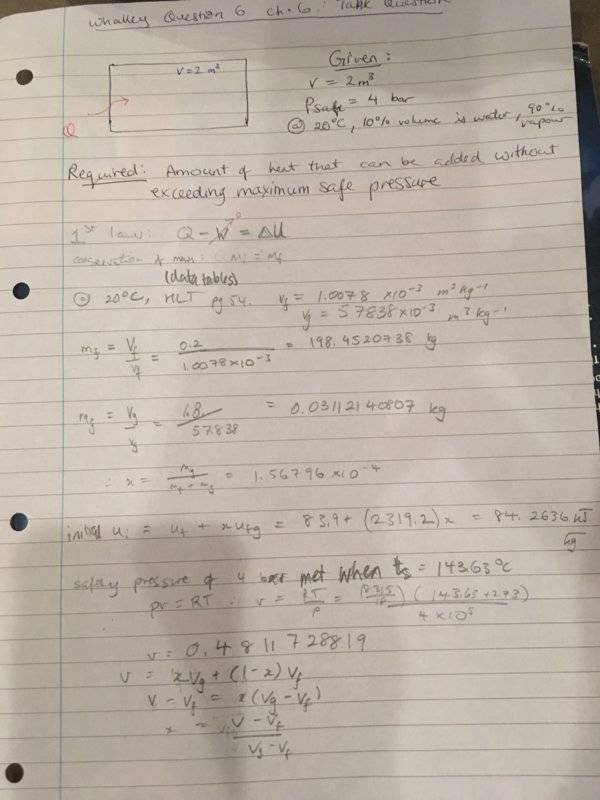# Thermodynamics of an Insulated Box: Water-Steam Question

Master1022

## Homework Statement

A sealed, thermally insulated tank of volume 2 $m^3$ has a safe working pressure of 4 bar. At 20 degrees Celcius, 10% of the volume is occupied by water, the remainder by water vapour. Calculate how much heat can be added without exceeding the safe working pressure.

This question is from "Basic Engineering Thermodynamics' - P. B. Whalley - p. 63, q6.6

## Homework Equations

1st law of Thermodynamics: $Q - W = \Delta U$
Other equations in the photo

## The Attempt at a Solution

The attached photo is my attempt (apologies, was trying to find out how to make it just an attachment). Hopefully, it is legible, was initially working in draft...My method outline:
1. work out the initial masses of liquid and vapour
2. work out the dryness fraction
3. work out the initial (specific) internal energy
4. using ideal gas law to calculate specific volume that would give pressure of 4 bar
5. calculate final dryness fraction
6. calculate final (specific) internal energy
7. find the difference between the U values (after including mass in the calculation)

However, in step 5, I am getting a dryness fraction > 1, which doesn't make sense. I would appreciate any help regarding any flaws in my method or working.